×
INTELLIGENT WORK FORUMS
FOR ENGINEERING PROFESSIONALS

Are you an
Engineering professional?
Join Eng-Tips Forums!
• Talk With Other Members
• Be Notified Of Responses
• Keyword Search
Favorite Forums
• Automated Signatures
• Best Of All, It's Free!

*Eng-Tips's functionality depends on members receiving e-mail. By joining you are opting in to receive e-mail.

#### Posting Guidelines

Promoting, selling, recruiting, coursework and thesis posting is forbidden.

# Log-Log plots (x_0 vs w) why slopes are -1 and -2 when system goes infinity in first and second syms

## Log-Log plots (x_0 vs w) why slopes are -1 and -2 when system goes infinity in first and second syms

(OP)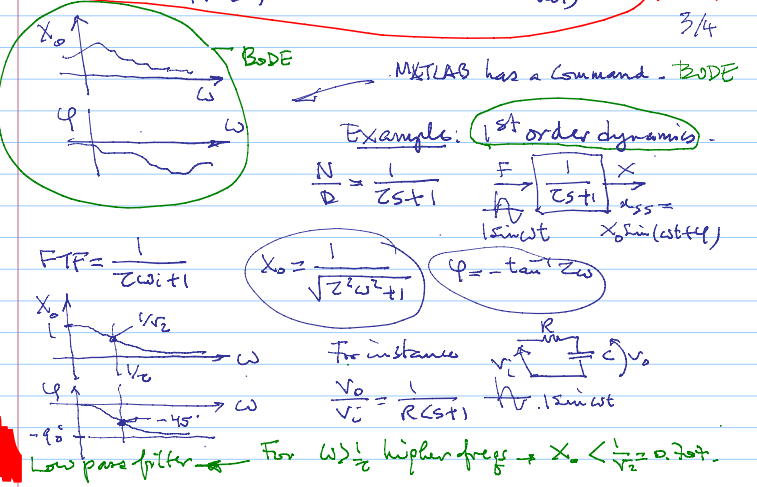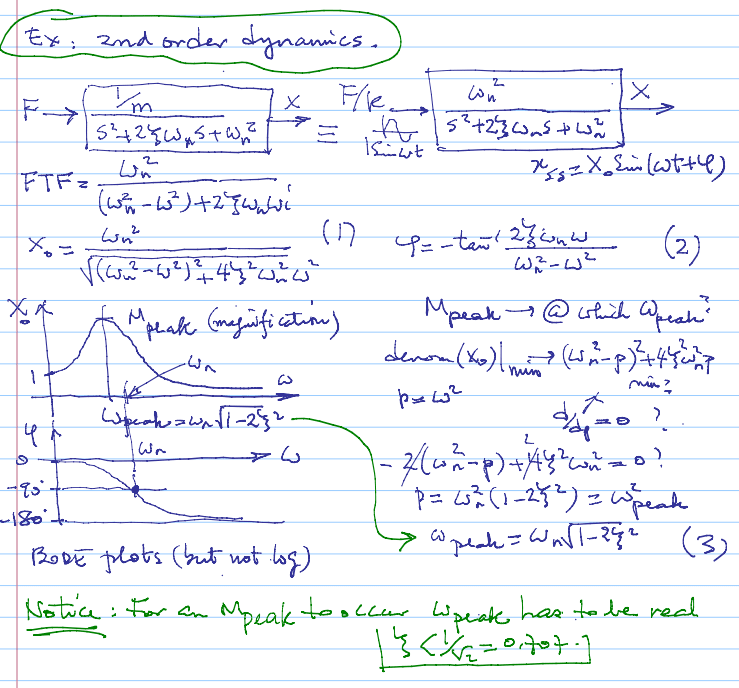Log-Log plots (x_0 vs w) why slopes are -1 and -2 when the system goes to infinity in first and second-order systems respectively.

### RE: Log-Log plots (x_0 vs w) why slopes are -1 and -2 when system goes infinity in first and second syms

(OP)
Both system equations and plots are provided in the attached files

### RE: Log-Log plots (x_0 vs w) why slopes are -1 and -2 when system goes infinity in first and second syms

(OP)
well, log is just used as a scale here, the main question is why the slopes are -1 and -2?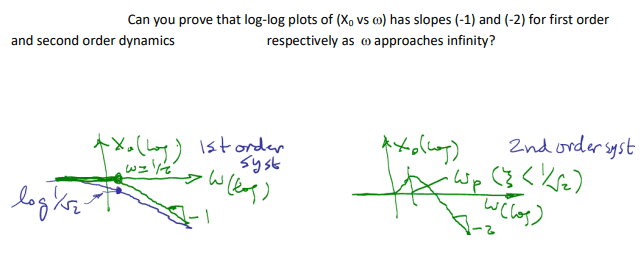### RE: Log-Log plots (x_0 vs w) why slopes are -1 and -2 when system goes infinity in first and second syms

#### Quote:

well, log is just used as a scale here,

NO!!!! Taking the log creates a linear equation. What is the slope of that equation?

TTFN (ta ta for now)
I can do absolutely anything. I'm an expert! https://www.youtube.com/watch?v=BKorP55Aqvg
FAQ731-376: Eng-Tips.com Forum Policies forum1529: Translation Assistance for Engineers Entire Forum list http://www.eng-tips.com/forumlist.cfm

### RE: Log-Log plots (x_0 vs w) why slopes are -1 and -2 when system goes infinity in first and second syms

(OP)
the slope is -1 and -2 for first and second-order systems respectively?

### RE: Log-Log plots (x_0 vs w) why slopes are -1 and -2 when system goes infinity in first and second syms

(OP)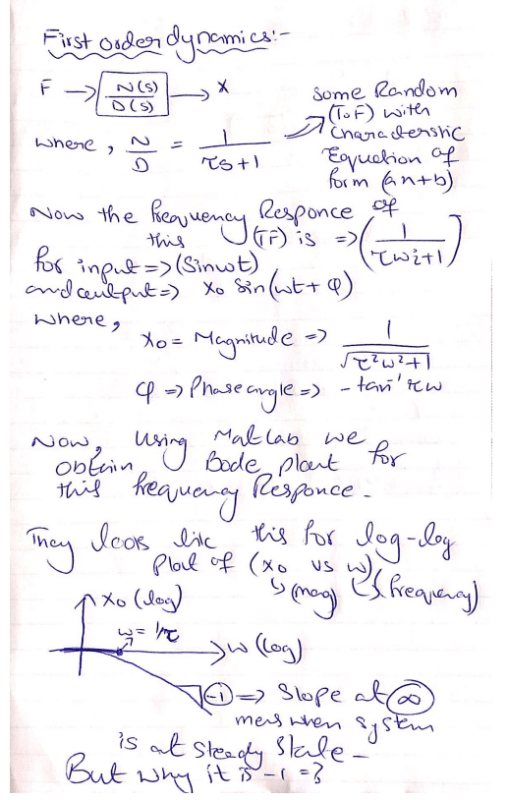this is what I can explain regarding first-order dynamics and the difference between this and second order is just the damping ratio is involved in the second-order characteristic equation and the highest degree is 2 and the plot you get for frequency response of the log-log plot of x vs w is already given in above pictures.

### RE: Log-Log plots (x_0 vs w) why slopes are -1 and -2 when system goes infinity in first and second syms

(OP)
I think as the ratio is constant for the change in vertical vs change in horizontal for 1 st order dynamics thus, we get a 1 slope, and the reason it's negative is the inverse relation between w and x.

### RE: Log-Log plots (x_0 vs w) why slopes are -1 and -2 when system goes infinity in first and second syms

You are not showing the final part.

What is the equation for a line?

How does that look like the logarithm of the equation of the transfer function? What assumptions do you need to make?

TTFN (ta ta for now)
I can do absolutely anything. I'm an expert! https://www.youtube.com/watch?v=BKorP55Aqvg
FAQ731-376: Eng-Tips.com Forum Policies forum1529: Translation Assistance for Engineers Entire Forum list http://www.eng-tips.com/forumlist.cfm

### RE: Log-Log plots (x_0 vs w) why slopes are -1 and -2 when system goes infinity in first and second syms

(OP)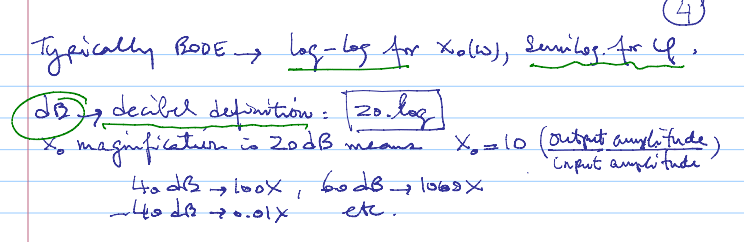### RE: Log-Log plots (x_0 vs w) why slopes are -1 and -2 when system goes infinity in first and second syms

(OP)
I don't think I have an equation that you ask for, but the above image might help.

### RE: Log-Log plots (x_0 vs w) why slopes are -1 and -2 when system goes infinity in first and second syms

(OP)
that is the general equation, we can have any value of taw in there. As in this image, the taw is RC, but the main idea is no matter what is the taw, the plots will remain the same at a steady state. with the slope of -1 for 1st order systems.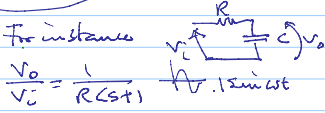### RE: Log-Log plots (x_0 vs w) why slopes are -1 and -2 when system goes infinity in first and second syms

(OP)
Well, if we put infinity instead of taw, the characteristic eq goes to infinity and the TF goes to 0.

### RE: Log-Log plots (x_0 vs w) why slopes are -1 and -2 when system goes infinity in first and second syms

(OP)
I cant see what A-line(Asymptotes) has to do with slope?

#### Red Flag This Post

Please let us know here why this post is inappropriate. Reasons such as off-topic, duplicates, flames, illegal, vulgar, or students posting their homework.

#### Red Flag Submitted

Thank you for helping keep Eng-Tips Forums free from inappropriate posts.
The Eng-Tips staff will check this out and take appropriate action.

#### Resources

Low-Volume Rapid Injection Molding With 3D Printed Molds
Learn methods and guidelines for using stereolithography (SLA) 3D printed molds in the injection molding process to lower costs and lead time. Discover how this hybrid manufacturing process enables on-demand mold fabrication to quickly produce small batches of thermoplastic parts. Download Now
Examine how the principles of DfAM upend many of the long-standing rules around manufacturability - allowing engineers and designers to place a partâ€™s function at the center of their design considerations. Download Now
Industry Perspective: Education and Metal 3D Printing
Metal 3D printing has rapidly emerged as a key technology in modern design and manufacturing, so itâ€™s critical educational institutions include it in their curricula to avoid leaving students at a disadvantage as they enter the workforce. Download Now

Close Box

# Join Eng-Tips® Today!

Join your peers on the Internet's largest technical engineering professional community.
It's easy to join and it's free.

Here's Why Members Love Eng-Tips Forums:

•Talk To Other Members
• Notification Of Responses To Questions
• Favorite Forums One Click Access
• Keyword Search Of All Posts, And More...

Register now while it's still free!